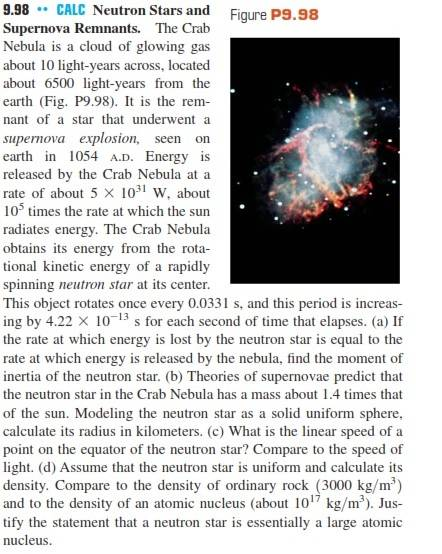# Moment of inertia of a neutron star

kent davidge

## Homework Statement## Homework Equations

rotational kinetic energy = 0.5 I ⋅ M ⋅ ω², where I is the moment of intertia, M the mass and ω the angular speed

## The Attempt at a Solution

T = period of revolution
K = kinetic energy associated with rotational moviment
Since T increases with time according the text,

T = ƒ(t) = 3.31(10^-2) + 1.40(10^-14)t
and I = 2K / ω²M = 2 ⋅ 5(10^31)t / [2π / [3.31(10^-2) + 1.40(10^-14)t]² ⋅ 1.4(1.99)(10^30)]

Last edited:

Mentor
rotational kinetic energy = 0.5 I ⋅ M ⋅ ω², where I is the moment of intertia, M the mass and ω the angular speed
Quick comment: Get the mass out of that formula (the mass is already accounted for in the moment of inertia): rot KE = 0.5 I ⋅ ω²

Mentor
Hint: The power will be equal to the rate of change of the rotational energy with respect to time. So think about differentiation of the rotational energy equation, taking into account that ω is a function of time.

kent davidge
Doc Al Ok.
gneill Would it becomes dk/dt = - 5 × (10^31) = - 4.22(10^-13)4π²⋅I / (4.22(10^-13)t + 0.0331)³ and for t = 0, I ≅ 1.09 × 10^25 ?

Mentor
Let's use symbols and avoid plugging in any numbers until the end.

The power: ##p = 5 ⋅ 10^{31}~W ##
The current period: ##\tau = 0.0331~s ##
The rate of change of the period: ##\frac{Δ\tau}{Δt} = -4.22⋅10^{-13} ##

Write the simple relationship for the angular speed ω given the period ##\tau##. Assume that ω and ##\tau## are functions of time, ##ω(t)## and ##\tau(t)##, and differentiate (hint: chain rule). You'll end up with ##\frac{d ω}{dt}## in terms of ##\tau## and ##\frac{d \tau}{dt}##. Note that a useful approximation is ##\frac{d \tau}{dt} ≈ \frac{Δ\tau}{Δt}##

I suspect that that should be sufficient hints...

kent davidge
Ok. I will do that.